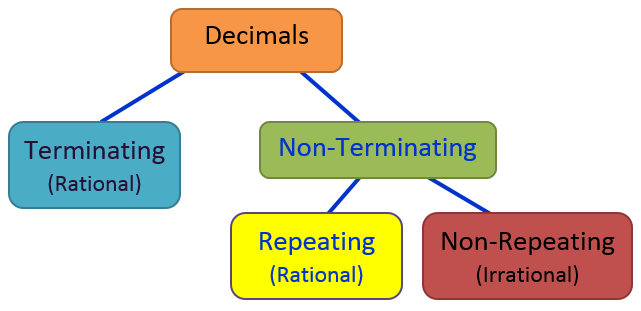# Decimals

A decimal is any number in our base-ten number system.

The position of the digit in the decimal number determines the digit’s value.Decimals show numbers that are in between two whole numbers. A decimal has two parts, the integer part and the fractional part, which starts with the dot character. Example:As an example of a decimal number, let’s take the number 65.894Sixty-five and eight hundred and ninety -four thousandths

The number after the decimal point can be collectively pronounced as 8 tenths, 9 hundredths and 4 thousandths or, more simply, as 894 thousandths.

Notice that all place values to the right of the decimal point end in ths.

The decimal point is read as “and“, to indicate the separation from the whole number and the decimal fraction parts.

Decimal Place Value Poem:

Reading Decimals is easy you’ll see,
They have two names like you and me.
First, you say the name as if there were no dot,

Then you say the name of the last place value spot!

### Decimal Numbers

Decimal values can be written as a decimal fraction (fractions with a denominator of 10, 100, 1000, …)Decimal Standard Form to Word Names:

0.8 = eight tenths

0.95 = ninety five hundredths

0.345 = three hundred forty five thousandths

Hint: When writing a decimal number that is less than 1, a zero is normally used in the one´s place.

Remember: Writing zeroes to the right of the decimal does not change its value. Example:

0.8 = 0.80 = 0.800

terminating decimal reaches an end after a finite number of digits.

Example:  1.5 is exactly equal to ‘one and one half’

A  Repeating decimal (non terminating) is a decimal that does not  end. Instead is goes on forever.

Example: 1/3 = 0.33333333…Rational Numbers includes integers, terminating decimals, and repeating decimals as well as fractions.

Create a graphic organizer (Frayer Model) for the following vocabulary words:  Decimal numbers, Standard form, Expanded form, Word form.